Algebra 2 5-8 Complete Lesson: Polynomial Models in the Real World
starstarstarstarstarstarstarstarstarstar
4.5 (1 rating)
by Matthew Richardson
| 26 Questions
Note from the author:
A complete formative lesson with embedded slideshow, mini lecture screencasts, checks for understanding, practice items, mixed review, and reflection. I create these assignments to supplement each lesson of Pearson's Common Core Edition Algebra 1, Algebra 2, and Geometry courses. See also mathquest.net and twitter.com/mathquestEDU.The outlined content above was added from outside of Formative.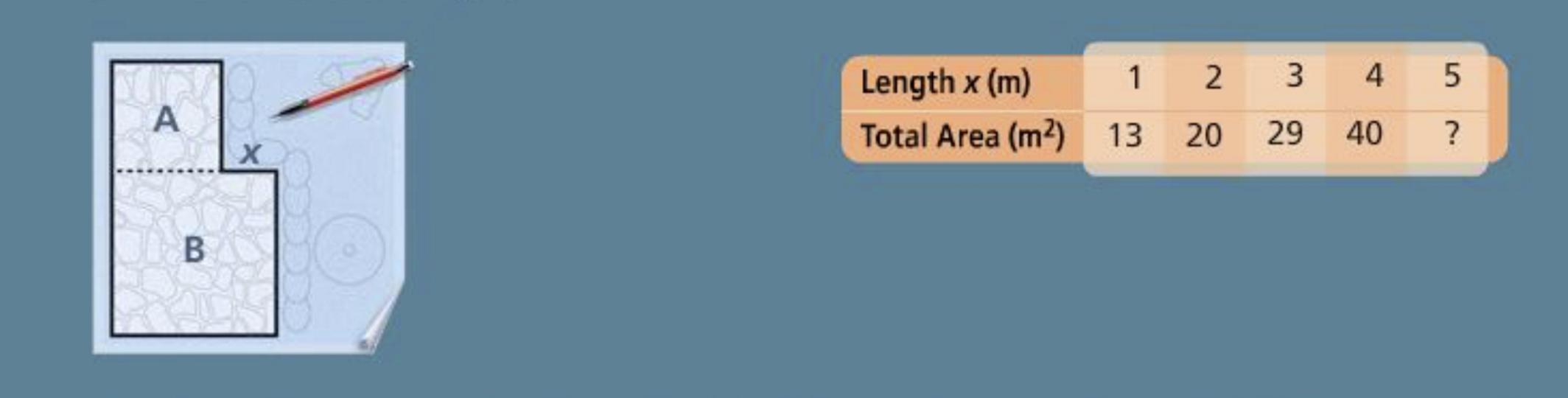a
b
1
2
You are designing a patio. Square A is where you will place your grill. You are experimenting with your design by varying the size of square B.
The table shows the total patio area for each of five different lengths x.
1
10 pts
Solve It! Based on the pattern in the table, find the total area when x is 5.
58 m²
53 m²
43 m²
60 m²
2
10 pts
Solve It! What type of polynomial function does the data fit? Explain.
The data fit a linear function because the first differences are constant.
The data fit a quadratic function because the second differences are constant and the first are not.
The data fit a cubic function because the third differences are constant and the second are not.
The data fit a quartic function because the fourth differences are constant and the third are not.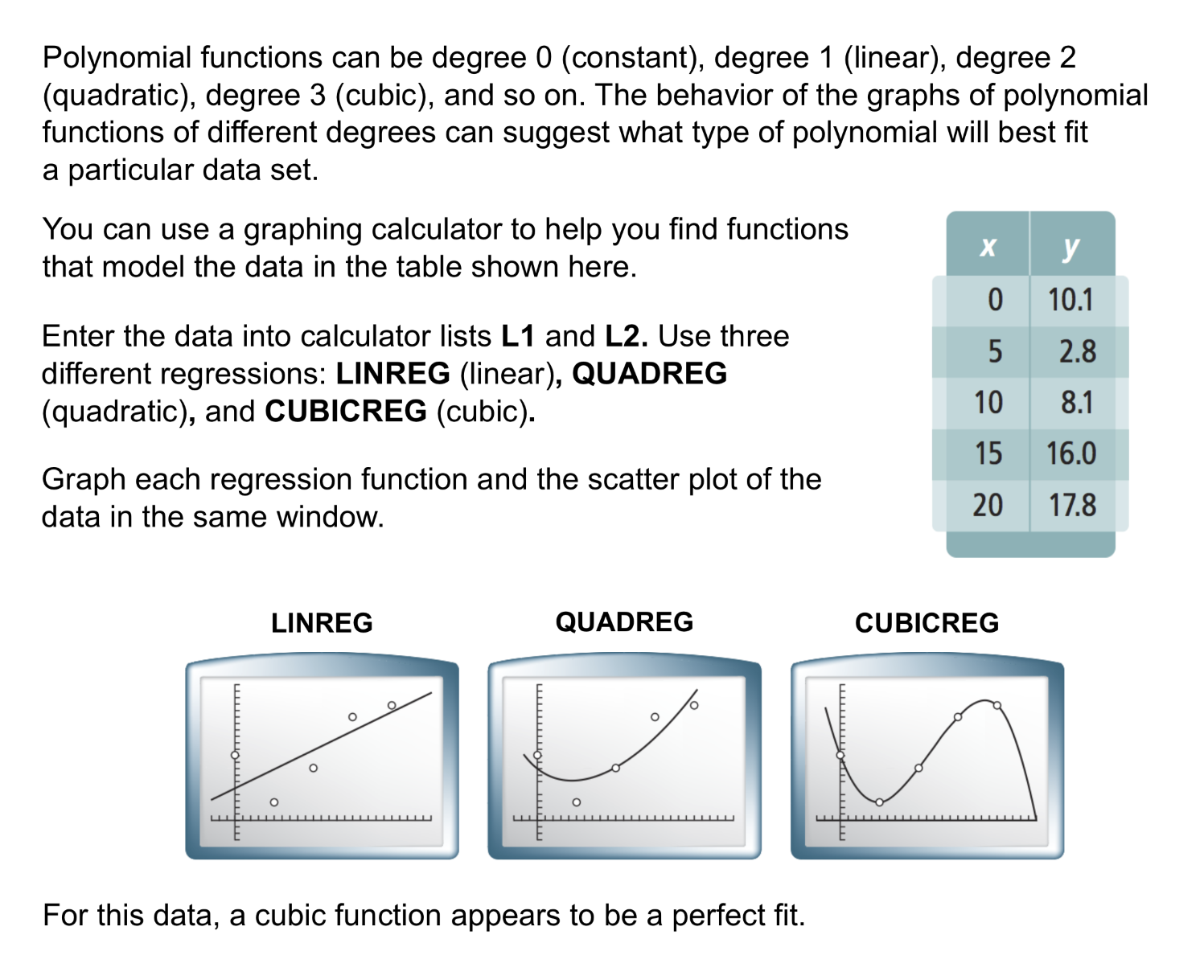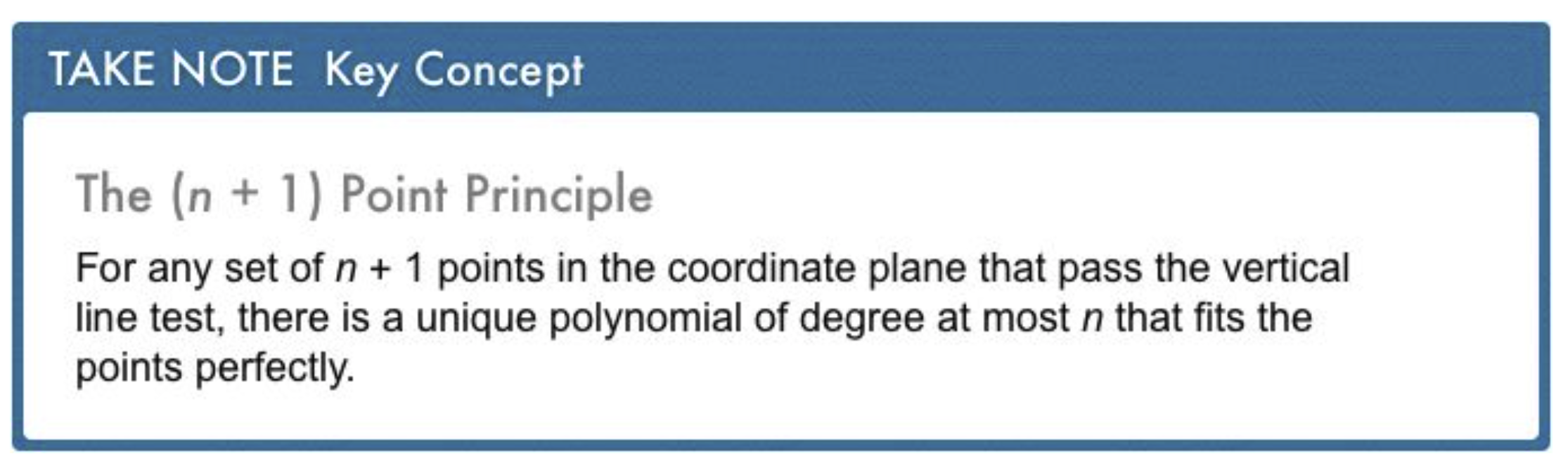3
5 pts
In Your Own Words: Restate the (n + 1) Point Principle in your own words.4
4
10 pts
Problem 1 Got It?
A
B
C
D5
5
10 pts
Problem 2 Got It?
A
B
C
D
6
10 pts
Problem 3 Got It? If four data points are given, which type of regression function guarantee ensure a perfect fit?
constant
linear
cubic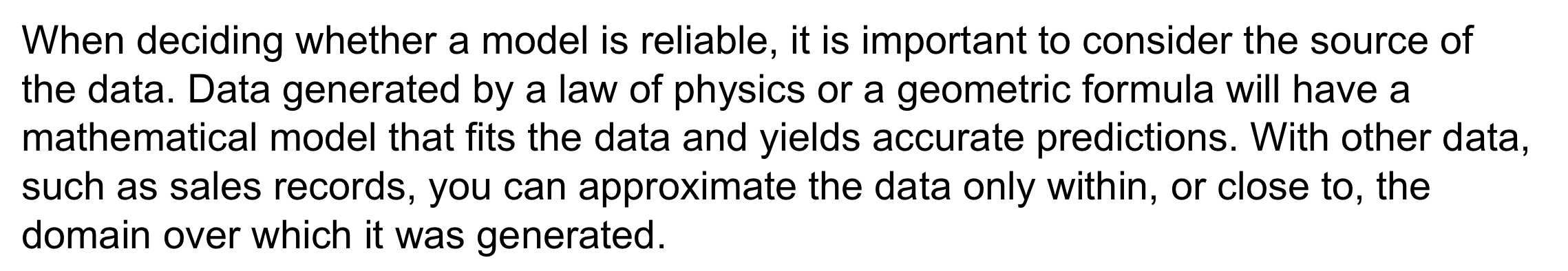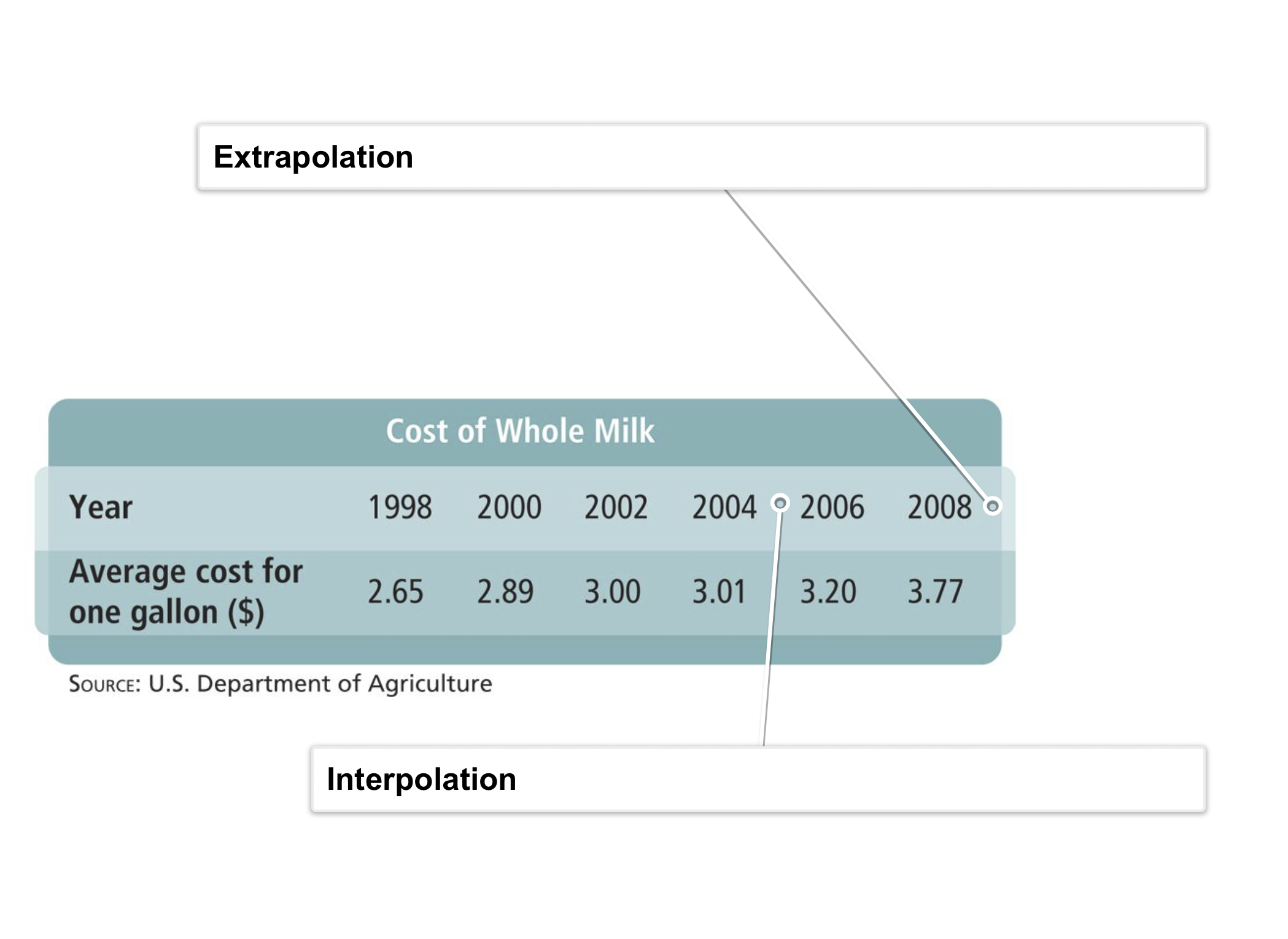7
30 pts
Problem 4 Got It? Create a table in the embedded Desmos utility and use it to find a linear regression model of the cheese consumption data. Let x = years since 1900.

Recall Desmos' linear regression notation: y1~ax1+b.

Zoom and pan your graph to establish an appropraite viewing window that contains all data points and the regression line.

8
10 pts
Problem 4 Got It? Use the model you created above to estimate cheese consumption for 1980, 2000, and 2012 algebraically and/or graphically. Remember that you let x represent years since 1900.
Also, identify the prediction years in which you can have the most and least confidence.
Hint: Consider interpolation vs extrapolation.
• 26.31 lb
• 23.07 lb
• 21.53 lb
• 17.7 lb
• 1980
• 2000
• 2012
• Estimated consumption in 1980
• Estimated consumption in 2000
• Estimated consumption in 2012
• Two prediction years with greatest confidence
• Prediction year with least confidence9
9
10 pts
A
B
C
D10
10
10 pts
A
B
C
D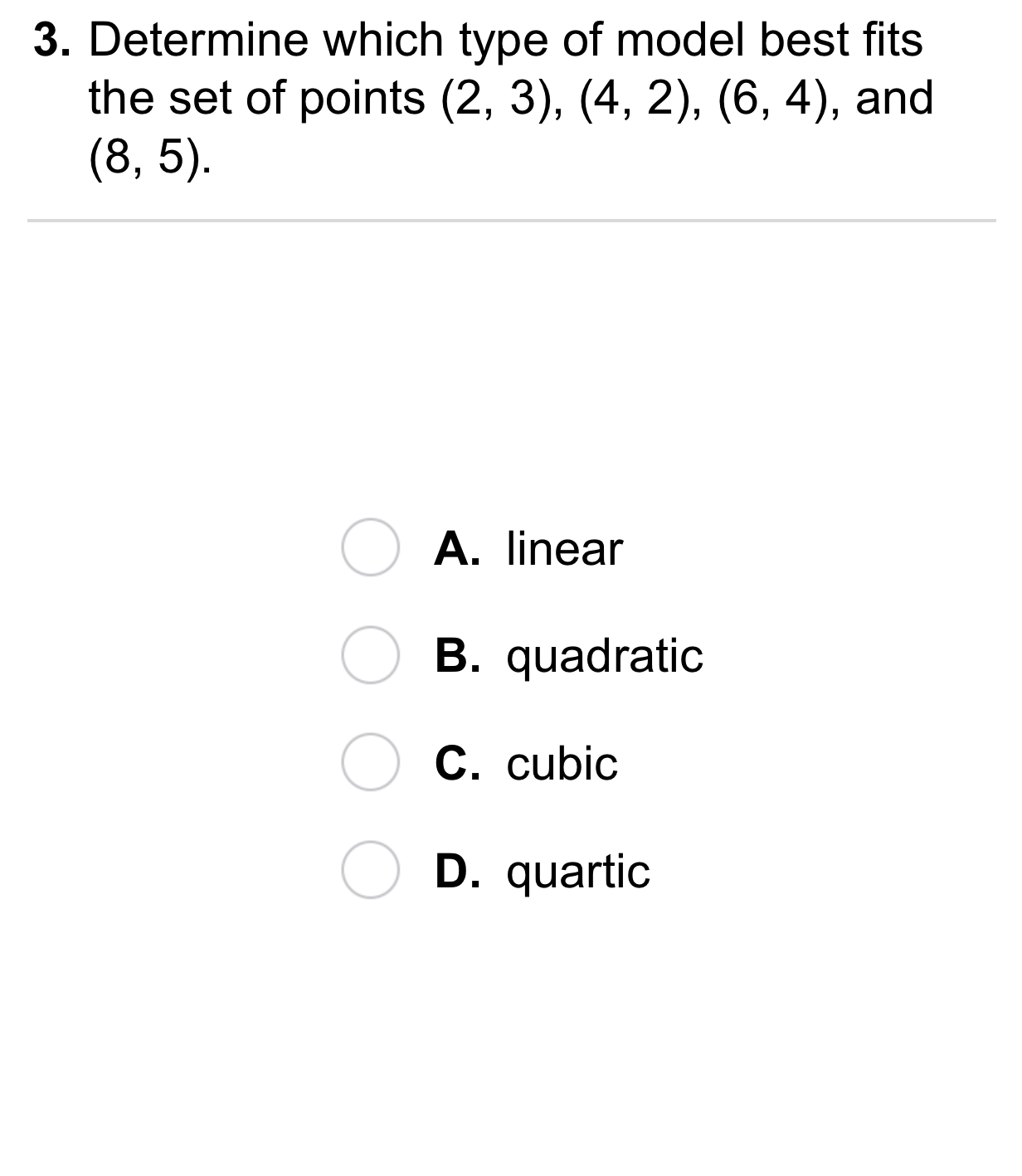11
11
10 pts
A
B
C
D12
12
10 pts
A
B
C
D13
10 pts
Vocabulary: Explain which form of estimation, interpolation or extrapolation, is more reliable.
Interpolation
Extrapolation
14
10 pts
Reasoning: Is it possible to create a cubic function that passes through (0, 0), (-1, 1), (-2, 2), and (-3, 9)? Explain.
15
10 pts
Writing: The value for a quartic model is 0.94561. The value for a cubic model of the same data is 0.99817. Which model seems to show a better fit? Explain.16
10 pts
Review Lesson 5-7: Expand the binomial.

17
10 pts
Review Lesson 5-7: Expand the binomial.

18
10 pts
Review Lesson 1-6: Write the compound inequality as an absolute value inequality.

19
10 pts
Review Lesson 1-6: Write the compound inequality as an absolute value inequality.

20
10 pts
Review Lesson 1-4: Solve the formula for the variable s.

21
10 pts
Review Lesson 1-4: Solve the formula for the variable r.

22
10 pts
Review Lesson 4-1: Graph the functions on the same coordinate plane. Zoom and pan your graph to establish an appropraite viewing window. Consider the similarities and differences between the graphs.23
10 pts
Vocabulary Review: Drag each item from the left column to its corresponding model in the right column.
• numerical data
• airplane
• house
• floor plan
• equation
• model airplane24
10 pts
Use Your Vocabulary: The points (1, 3) and (2, 6) are collinear. Extrapolate the y-value of the point on the same line when x = 4.

Fill in the blank: The point (4, __?__) is also on the line.

4
10
6
12
25
100 pts
Notes: Take a clear picture or screenshot of your Cornell notes for this lesson. Upload it to the canvas. Zoom and pan as needed.

For a refresher on the Cornell note-taking system, click here.
26
10 pts
Reflection: Math Success
Add to my formatives list

Formative uses cookies to allow us to better understand how the site is used. By continuing to use this site, you consent to the Terms of Service and Privacy Policy.